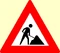This article is still under constructionPlease post comments, questions and suggestions on the talk page, not in the article itself. Thank you.The horse paradox is an apparent paradox which arises from the following supposed demonstration, using mathematical induction, of the statement All horses are the same colour:

As the basis case, note that in a set containing a single horse, all horses are clearly the same colour. Now assume the truth of the statement for all sets of at most n horses.

Let there be n + 1 horses in a set. Remove the first horse to get a set of n horses. By the induction hypothesis, all horses in this set are the same colour.

It remains to show that this colour is the same as that of the horse we removed. But this is easy: put back the first horse, take out a different horse and apply the induction principle to this set of n horses. Thus all horses in any set of n + 1 horses are the same colour. By the principle of induction, we have established that all horses are the same colour.

## Explanation

The horse paradox makes the implicit assumption that the two subsets of horses to which we apply the induction assumption have a common element. But this fails when n = 1.

Indeed, let the two horses be horse A and horse B. When you remove horse A, it is true that the remaining horses in the set are the same color (only horse B remains.) If you put horse A back and take out horse B, you are left with a new set of just horse A, which may or may not be the same color as horse B. The problem occurs when you try to assume that the two sets have the same common properties. There are no common elements (horses) between the two sets, therefore it is unknown if the two horses share the same property.

Thus it is not truly a paradox, but merely the result of flawed reasoning. The horse paradox exposes the pitfalls arising from failure to consider special cases for which a general statement may be false.